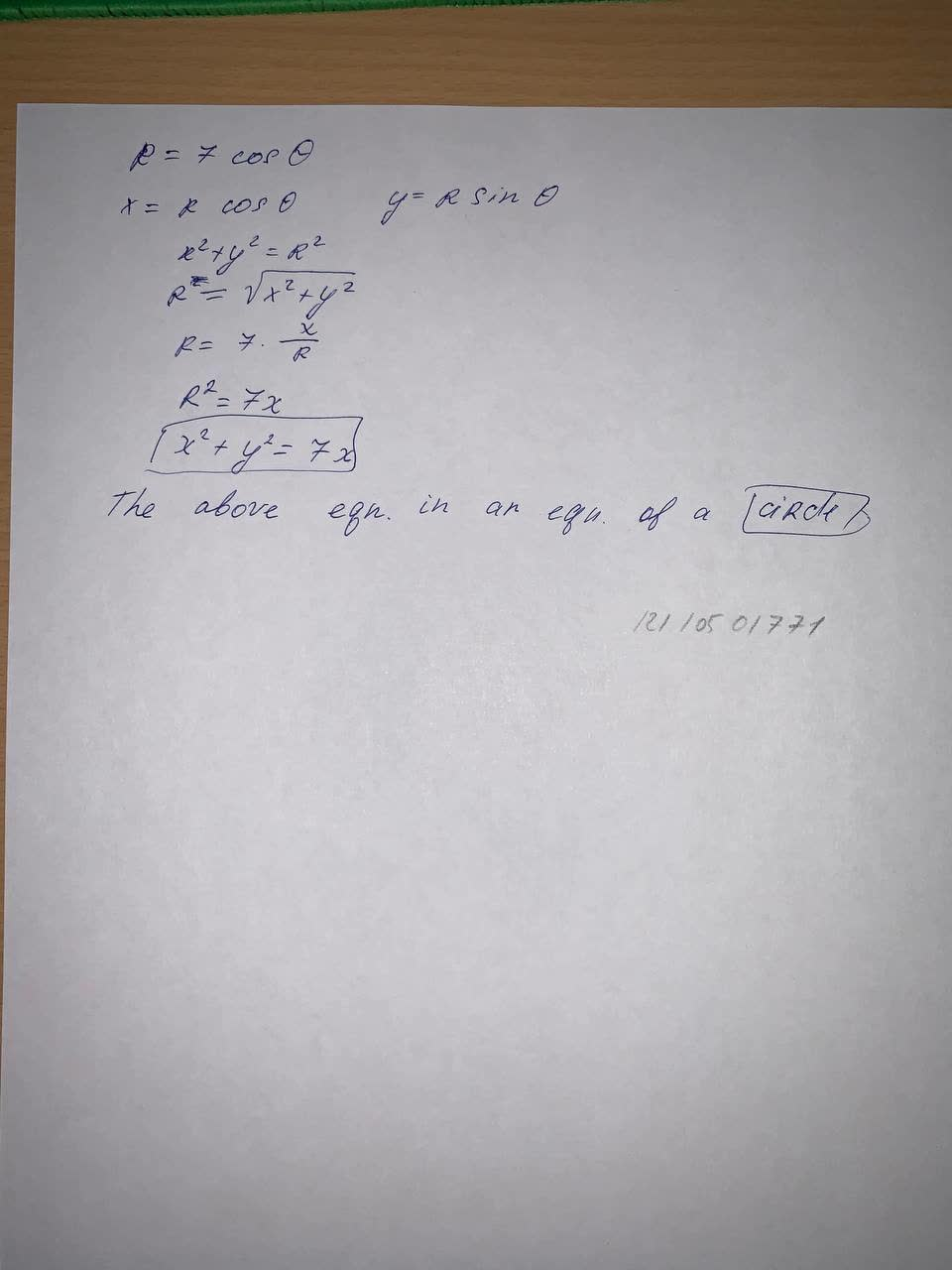Question# Find a Cartesian equation for the curve and identify it. r = 7 \cos (\theta)

Integrals
ANSWERED$$r = 7 \cos (\theta)$$